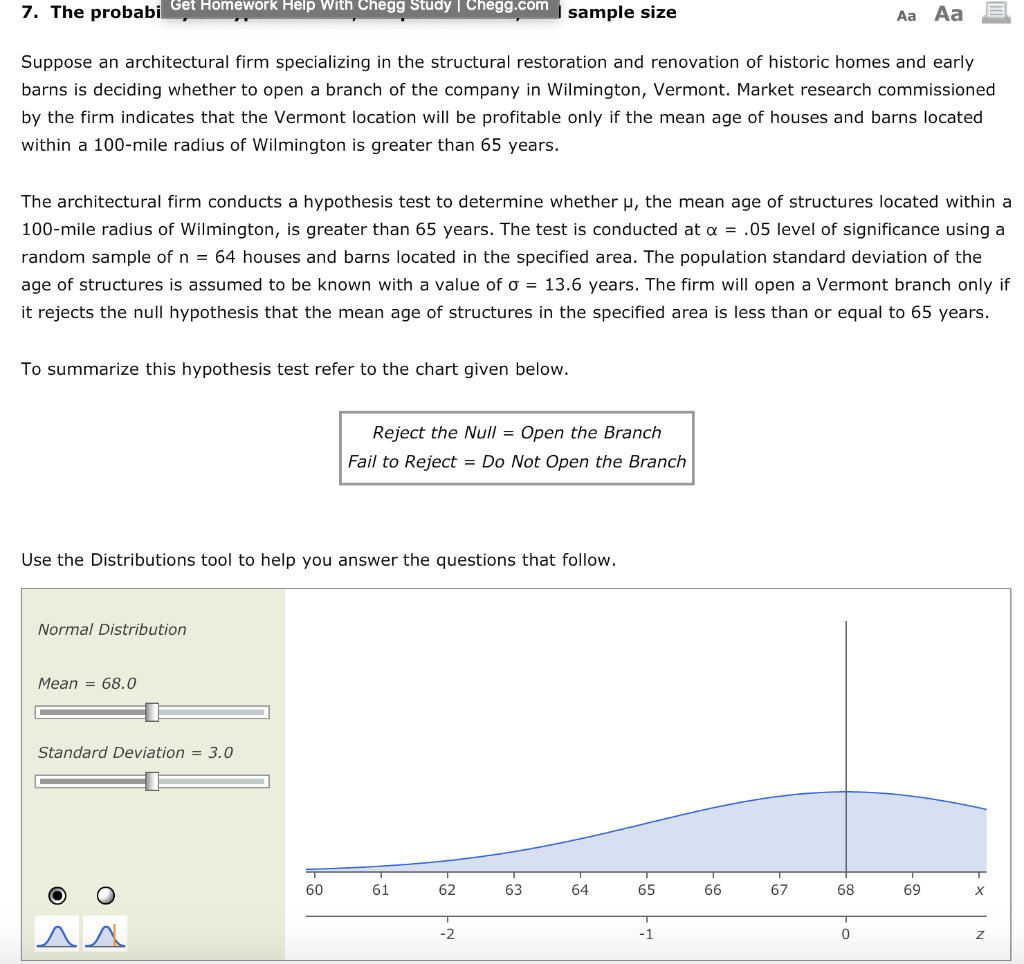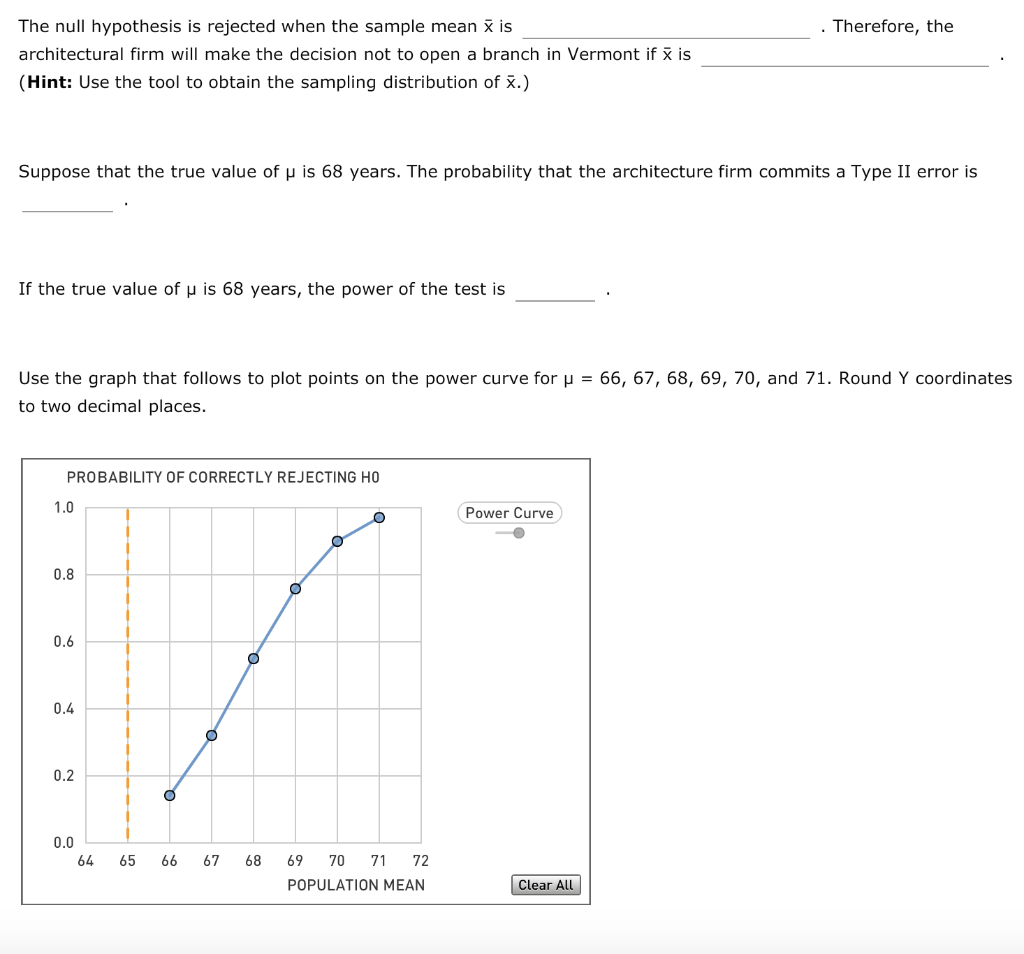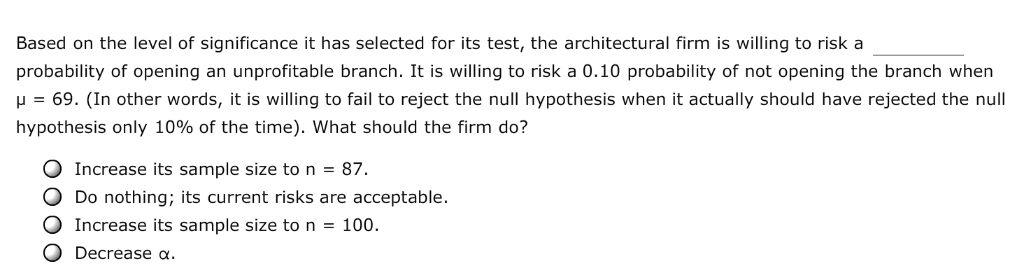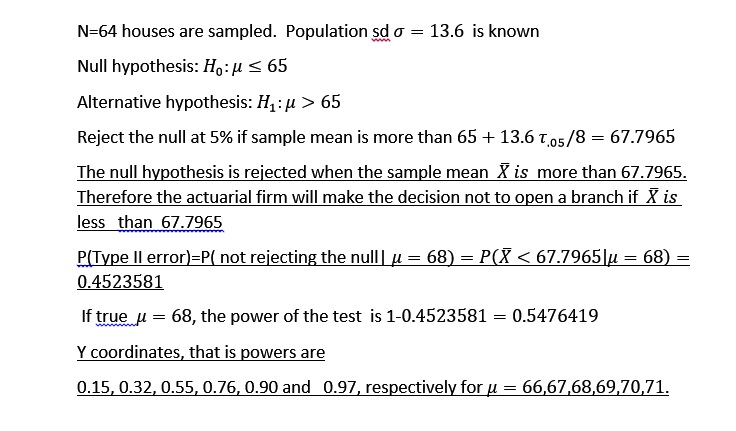# 7. The probabi Get Homework Help With Chegg Study Chegg.com sample size Aa Aa Suppose an architec...7. The probabi Get Homework Help With Chegg Study Chegg.com sample size Aa Aa Suppose an architectural firm specializing in the structural restoration and renovation of historic homes and early barns is deciding whether to open a branch of the company in Wilmington, Vermont. Market research commissioned by the firm indicates that the Vermont location will be profitable only if the mean age of houses and barns located within a 100-mile radius of Wilmington is greater than 65 years. The architectural firm conducts a hypothesis test to determine whether μ, the mean age of structures located within a 100-mile radius of Wilmington, is greater than 65 years. The test is conducted at α = .05 level of significance using a random sample of n - 64 houses and barns located in the specified area. The population standard deviation of the age of structures is assumed to be known with a value of σ = 13.6 years. The firm will open a Vermont branch only if it rejects the null hypothesis that the mean age of structures in the specified area is less than or equal to 65 years To summarize this hypothesis test refer to the chart given below Reject the Null-Open the Branch Fail to Reject Do Not Open the Branch Use the Distributions tool to help you answer the questions that follow Normal Distribution Mean68.0 Standard Deviation3.0 60 61 62 63 64 65 67 68 69
The null hypothesis is rejected when the sample mean X is architectural firm will make the decision not to open a branch in Vermont if Hint: Use the tool to obtain the sampling distribution of X.) Therefore, the is Suppose that the true value of μ is 68 years. The probability that the architecture firm commits a Type II error is If the true value of μ is 68 years, the power of the test is Use the graph that follows to plot points on the power curve for to two decimal places 66, 67, 68, 69, 70, and 71. Round Y coordinates PROBABILITY OF CORRECTLY REJECTING HO Power Curve 1.0 0.8 0.6 0.4 0.2 0.0 64 65 66 67 68 69 70 71 72 POPULATION MEAN Clear All
Based on the level of significance it has selected for its test, the architectural firm is willing to risk a probability of opening an unprofitable branch. It is willing to risk a 0.10 probability of not opening the branch when μ-69. (in other words, it is willing to fail to reject the null hypothesis when it actually should have rejected the null hypothesis only 10% of the time), what should the firm do? Increase its sample size to n 87. O Do nothing; its current risks are acceptable. O Increase its sample size to n 0 Decrease α 100.##### Add Answer of: 7. The probabi Get Homework Help With Chegg Study Chegg.com sample size Aa Aa Suppose an architec...
More Homework Help Questions Additional questions in this topic.